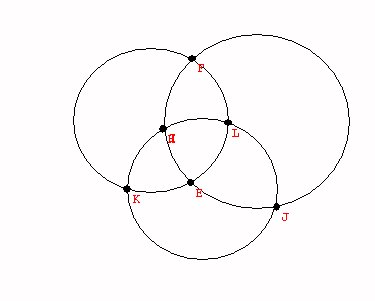Tuesday,  November 18

Last class
Desargues' Theorem in the plane:
Duality again!

• Desargues 10-10 Configuration:
•Planar Configuration
•
 3 points on each line 3 lines through each point Triangles in Perspective  from a point Triangles in Perpective from a line

• Pascal Configuration and [the dual] Brianchon Configuration.
•Pascal: 9 points - 7 lines A Point Conic- The conic is described by a set of pointsBrianchon: 9 lines - 7 points A Line Conic-The conic is described by a set of lines

Pascal's Theorem - Brianchon's Theorem.
Show video on projective generation of conics.
This showed how to construct a conic using Pascal's Theorem and it converse.

Activity on Pascal's theorem.

Comment about the Principle of Duality in Projective geometry:
If you can justify a result of the form ...."line"...."point"....
then the same result will be true if you switch the words line and point appropriately. ...."point"...."line"....

This principle works because the key properties off the projective geometry satisfy this quality. E.g. "Two points determine a unique line"  corresponds to "Two lines determine a unique point" which is also true in projective geometry, but not in Euclidean (flatland) geometry.

Another example of Duality in Projective Geometry:

 3 points on each line 3 lines through each point Triangles in Perspective  from a point Triangles in Perpective from a line

Desargues Theorem and its Dual.P: If triangle ABC is Perspective with triangle A'B'C' with center of perpective O then the P-points of intersection of AB, A'B'; AC,A'C'; BC,B'C' all lie on the same P-line.

P':If triangle abc is Perspective with triangle a'b'c' with axis of perpective o then the P-lines joining of a, a'; b,b'; c,c' all pass through  on the same P- point.

Notice: P' is also the converse of P!

Curves and Surfaces:What is possible? what is not?

What is the difference between Euclidean Geometry, Projective Geometry, and "Topology"

Euclid: congruence, similar... measurements, scale Questions: Is a triangle congruent/similar to another triangle?
Is an ellipse congruent /similar to a parabola?

Is a triangle congruent/similar to a square?

Projective: perspectivity, projectivity, lines, points of intersection and contact (tangency).
Questions: Is a triangle projectively related to another triangle?
Is an ellipse projectively related to a parabola?
Is a triangle
projectively related to a square?

Topology: "Bounded", "closed", "open", "connected"
Questions: Is a triangle topologically related to another triangle?
Is an ellipse
topologically related to a parabola?
Is a triangle
topologically related to a square?

Topology: Counting on a line.  Counting on a curve.
Counting in the plane. Counting on a surface.V+R = E + 2